### Home > CALC > Chapter 5 > Lesson 5.5.2 > Problem5-167

5-167.
1. Determine the following limits. For each, describe your method. Homework Help ✎

1.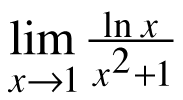2.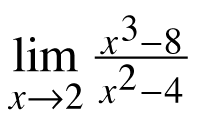3.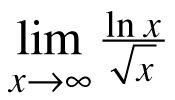4.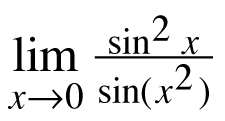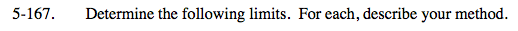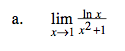The denominator does not equal 0. Evaluate.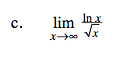$\text{Think about the graphs of }y=\text{ln}x\text{ and }y=\sqrt{x}.$

Which grows faster?

Is there a horizontal asymptote?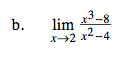x3 −8 = (x − 2)(x2 + 2x + 4)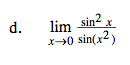Use two iterations of l'Hôpital's Rule.

1